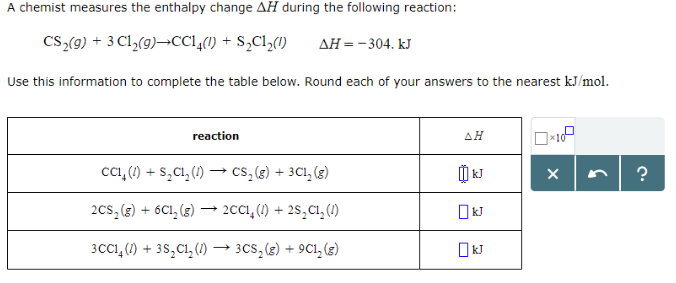# Problem: A chemist measures the enthalpy change ΔH during the following reaction: CS2 (g) + 3Cl2 (g) → CCl4 (l) + S2Cl2 (l)     ΔH = -304. kJ Use this information to complete the table below. Round each of your answers to the nearest kJ/mol.

###### FREE Expert Solution
81% (153 ratings)
###### FREE Expert Solution
81% (153 ratings)###### Problem Details

A chemist measures the enthalpy change ΔH during the following reaction:

CS2 (g) + 3Cl2 (g) → CCl4 (l) + S2Cl2 (l)     ΔH = -304. kJ

Use this information to complete the table below. Round each of your answers to the nearest kJ/mol.